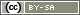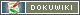This translation is older than the original page and might be outdated. See what has changed.

# Individual Feedback for Participants

Using the functions `value()`, `valueMean()`, the participant's answers can already be evaluated during the Questionnaire session (also see Score Responses). Some questions, like for instance Implicit association test (IAT), deliver an evaluation directly. Additionally, with the help of `statistic()` function, it is possible to evaluate values of all the previously tested participants.

Note: This tutorial shows how feedback can be displayed within or at the end of a questionnaire. Using the additional automatic data analysis module, it is possible to provide a PDF with a personal feedback for download, see Automated Result Reports.

Note: Instructions on how to give feedback on individual questions (correct/incorrect) can be found in a separate tutorial: Direct Feedback for Respondents

Note: Another tutorial explains how to provide a print view of a participant's own answers (“print/save my questionnaire”): `answersPrint()`

## Presenting outcome as text

It is easy to present the evaluation outcome as text. The `sprintf()` function makes it easy to format decimal values. Using `sprintf('%1.2f', \$value)` the decimal value `\$value` is formated in such a way so that there are 2 digits after after the point (two decimal digits).

### Single Values (I)

The easiest way to output single values is to use `html()`. Strings can be bound using a point (`.`) (for instance when binding fragments of HTML code and the output values that should be displayed).

```\$value = valueMean('AB01');
html('
<p>You evaluated the current TV programm on a scale from 1 to 10
and your average evaluation was '.sprintf('%1.1f', \$value).'.
Average evaluation for this program countrywide is 6.3</p>
');```

### Single Values (II)

Possibly, one may also want to output different lines of text depending on the outcome of the evaluation. To set this up the function Filter can be used:

```// Calculating the outcome value
\$value = valueMean('AB01_01', 'AB01_05', 'AB01_09');
// z-Transformation
\$zValue = (\$value - 5) / 2.8;
// Showing the appropriate text based on the outcome value
if (\$value < 0) {
// no valid data in \$value - no output
} elseif (\$zValue < -2) {
text('resultExtraSD-2');
} elseif (\$zValue < -1) {
text('resultExtraSD-1');
} elseif (\$zValue <= 1) {
text('resultExtraSD-0');
} elseif (\$zValue < 2) {
text('resultExtraSD+1');
} else {
text('resultExtraSD+2');
}```

### Multiple Values

Multiple values are commonly displayed in a table. The easiest way to do this is with text-blocks and placeholders. For instance you could save the following HTML code under Textblocks and Labels inside a new textblock results (“HTML code” representation).

```<table cellspacing="5" cellpadding="0" border="0">
<tr>
<th>Trait</th>
<th>Value of comparison</th>
</tr>
<tr>
<td>Extraversion</td>
<td>%val-extra%</td>
<td>3.2</td>
</tr>
<tr>
<td>Neuroticism</td>
<td>%val-neuro%</td>
<td>2.4</td>
</tr>
</table>```

The placeholders can finally directly be indicated in the command `text()`. Alternatively, `replace()` can also be used.

```text('result', array(
'%val-extra%' => valueMean('AB01_01', 'AB01_05', 'AB01_09'),
'%val-neuro%' => valueMean('AB01_02', 'AB01_06', 'AB01_10')
));```

## Visual Presentation

There are several different options available to present outcomes visually.

### Single Values

For single values (for example an IAT score) an HTML code can be used to show a scale with a mark on it.Save the following HTML code as a text in the list fo questions (the following examples assume that it gets the ID “RS01”).

```<!-- Heading for the figure -->
<div class="title" style="text-align: center">%title%</div>

<div style="position: relative">
<!-- The scale lies in the background -->
<div style="margin: 0 auto; width: 400px; height: 25px; background-image:url('ofb://slider.scale.sd-blue400S')">
<!-- In order to place the Marking on the scale, a unit (from 0 to 1) is placed as a block element.-->
<div style="position: absolute; top: -2px; left: 50%; width: 65px; height: 27px">
<!-- The actual Marking is going to be inserted at the above defined right place -->
<img src="ofb://slider.button.rhomb" alt="" style="position: absolute; left: %zValue100%%; margin-left: -8px" />
</div>
</div>
</div>```

The HTML code contains two placeholders: `%title%` and `%zValue100%`. It is designed to show a z-value between -3 and +3 and can be used as follows:

```// Determine the outcome value
\$value = valueMean('AB01_01', 'AB01_05', 'AB01_09');
// z-Transformation
\$zValue = (\$value - 5) / 2.8;
// Filter extreme values
if (\$zValue < -3) {
\$zValue = -3;
}
if (\$zValue > 3) {
\$zValue = 3;
}
// Use textblocks (only if \$value delivered a valid value)
if (\$value > 0) {
show('RS01', array(
'%title%' => 'Extraversion',
'%zValue%' => (string)round(\$zValue * 100)
));
}```

### Multiple Values (I)

Using the function `diagram2()`, one can place desired Diagrams into the Questionnaire. To learn how to use it please refer to the documentation of the function. An optimized function `chart()` is going to be available in future versions of SoSci Survey.

### Multiple Values (II)

You can use the ChartJS library to display nifty diagrams in your questionnaire. If the category labels are saved in the array `\$labels`, the results are saved in the array `\$ownData` and reference data is saved in the array `\$refData`, you can use the following text (in the example it has the identifier “CH01”, as DarstellungHTML_Code”) and the associated PHP code to display a bar chart. You can find clarifications and details at ChartJS Documentation.

```<!-- Container for the diagram -->
<canvas id="myChart" width="400" height="300"></canvas>

<script type="text/javascript">
<!--

var chartCanvas = document.getElementById('myChart').getContext('2d');
var chart01 = new Chart(chartCanvas, {
type: 'line',
data: {
labels: %labels%,
datasets: [{
label: 'Ihr Wert',
data: %ownData%,
backgroundColor: '#FF9900',
borderColor: '#FFCCAA',
}, {
label: 'Durchschnitt',
data: %refData%,
backgroundColor: '#AAAAAA',
borderColor: '#DDDDDD',
}]
},
options: {
indexAxis: 'y',
scales: {
x: {
beginAtZero: true
}
}
}
});

// -->
</script>```
```// Make ChartJS library available
library('ChartJS');

// Test data (here you would read data from the dataset)
\$labels = ['Motivation', 'Engagement', 'Qualifikation'];
\$ownData = [57, 42, 80];
\$refData = [52, 48, 74];

// Include text module with the HTML code for the chart
show('TX09', [
'%labels%' => json_encode(\$labels),
'%ownData%' => json_encode(\$ownData),
'%refData%' => json_encode(\$refData)
]);```